• ## 神经网络中的单层神经网络

千次阅读 多人点赞 2019-12-30 20:35:30
单层神经网络2.1 感知器2.2 数学描述2.3 感知器分类效果2.4 单层神经网络表示2.5 单层神经网络训练算法2.6 单层神经网络中的计算公式表示 文章综合一下几位大佬的文章： 杨强AT南京: DL01-6: 单层神经网络 企鹅号 ...


文章目录
一、神经网络1.概述1.1 结构1.2 神经元模型使用

2. 单层神经网络2.1 感知器2.2 数学描述2.3 感知器分类效果2.4 单层神经网络表示2.5 单层神经网络训练算法2.6 单层神经网络中的计算公式表示

文章综合一下几位大佬的文章：

杨强AT南京: DL01-6: 单层神经网络 企鹅号 - 轨道车辆: 技术篇：单层神经网络是什么，看完这篇文章你就懂了

一、神经网络
1.概述
神经网络是一种模拟人脑的神经网络以期能够实现类人工智能的机器学习技术。人脑中的神经网络是一个非常复杂的组织。成人的大脑中估计有1000亿个神经元之多。
看一个经典的神经网络。这是一个包含三个层次的神经网络。 🔹 红色的是输入层，绿色的是输出层，紫色的是中间层（也叫隐藏层）。 🔹 输入层有3个输入单元，隐藏层有4个单元，输出层有2个单元。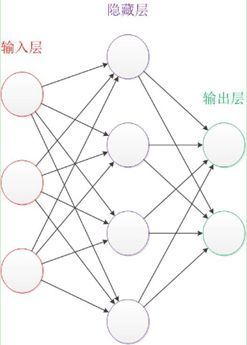1.1 结构
神经元模型是一个包含输入，输出与计算功能的模型。输入可以类比为神经元的树突，而输出可以类比为神经元的轴突，计算则可以类比为细胞核。
📢 设计一个神经网络时，输入层与输出层的节点数往往是固定的，中间层则可以自由指定；
📢 神经网络结构图中的拓扑与箭头代表着预测过程时数据的流向，跟训练时的数据流有一定的区别；
📢 结构图里的关键不是圆圈（代表“神经元”），而是连接线（代表“神经元”之间的连接）。每个连接线对应一个不同的权重（其值称为权值），这是需要训练得到的。
下图是一个典型的神经元模型：包含有3个输入，1个输出，以及2个计算功能。

注意中间的箭头线。这些线称为“连接”。每个上有一个“权值”。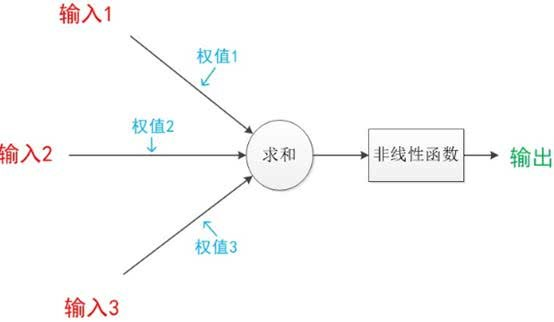📢 连接是神经元中最重要的东西。每一个连接上都有一个权重。

一个神经网络的训练算法就是让权重的值调整到最佳，以使得整个网络的预测效果最好。

我们使用a来表示输入，用w来表示权值。一个表示连接的有向箭头可以这样理解：在初端，传递的信号大小仍然是a，端中间有加权参数w，经过这个加权后的信号会变成aw，因此在连接的末端，信号的大小就变成了aw。
在其他绘图模型里，有向箭头可能表示的是值的不变传递。而在神经元模型里，每个有向箭头表示的是值的加权传递。如果我们将神经元图中的所有变量用符号表示，并且写出输出的计算公式的话，就是下图。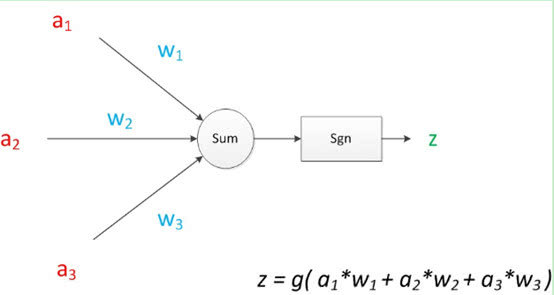1.2 神经元模型使用
我们有一个数据，称之为样本。样本有四个属性，其中三个属性已知，一个属性未知。我们需要做的就是通过三个已知属性预测未知属性。
具体办法就是使用神经元的公式进行计算。三个已知属性的值是a1，a2，a3，未知属性的值是z。z可以通过公式计算出来。
这里，已知的属性称之为特征，未知的属性称之为目标。假设特征与目标之间确实是线性关系，并且我们已经得到表示这个关系的权值w1，w2，w3。那么，我们就可以通过神经元模型预测新样本的目标。
2. 单层神经网络
2.1 感知器

来自百度百科：感知器 （神经网络模型）

1958年，计算科学家Rosenblatt提出了由两层神经元组成的神经网络。他给它起了一个名字–“感知器”（Perceptron），也可翻译为感知机。

神经细胞

感知器是生物神经细胞的简单抽象，神经细胞结构大致可分为：树突、突触、细胞体及轴突。 单个神经细胞可被视为一种只有两种状态的机器——激动时为‘是’，而未激动时为‘否’。神经细胞的状态取决于从其它的神经细胞收到的输入信号量，及突触的强度（抑制或加强）。当信号量总和超过了某个阈值时，细胞体就会激动，产生电脉冲。电脉冲沿着轴突并通过突触传递到其它神经元。 为了模拟神经细胞行为，与之对应的感知机基础概念被提出，如权量（突触）、偏置（阈值）及激活函数（细胞体）。

在人工神经网络领域中，感知器作为一种线性分类器，也被指为单层的人工神经网络，以区别于较复杂的多层感知器（Multilayer Perceptron）。
📢 在“感知器”中，有两个层次:
输入层 输入层里的“输入单元”只负责传输数据，不做计算。输出层 输出层里的“输出单元”则需要对前面一层的输入进行计算。
把需要计算的层次称之为“计算层”，并把拥有一个计算层的网络称之为“单层神经网络”。有一些文献会按照网络拥有的层数来命名，例如把“感知器”称为两层神经网络。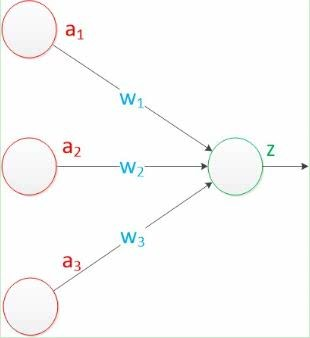假如我们要预测的目标不再是一个值，而是一个向量，例如[x,y]。那么可以在输出层再增加一个“输出单元”,如图：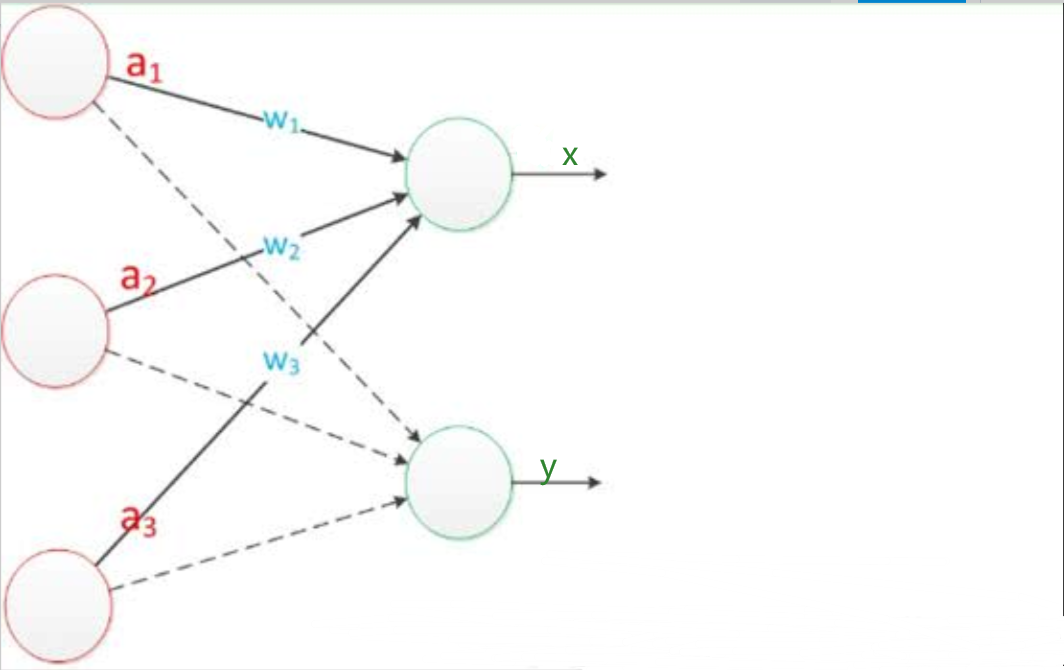2.2 数学描述
感知器使用特征向量来表示的前馈式人工神经网络，它是一种二元分类器，把矩阵上的输入（实数值向量）映射到输出值

f

(

x

)

f(x)

上（一个二元值）。

f

(

x

)

=

{

1

,

i

f

w

∗

x

+

b

>

0

0

,

e

l

s

e

f(x)= \begin{cases}1,\ \ \ if \ \ w*x+b>0 \\ 0, \ \ \ else \end{cases}

w

w

是实数的表权重的向量，

w

∗

x

w*x

是点积。

b

b

是偏置，一个不依赖于任何输入值的常数。偏置可以认为是激励函数的偏移量，或者给神经元一个基础活跃等级。

f

(

x

)

f(x)

(0 或 1)用于分类，判断是肯定的还是否定的，这属于二元分类问题。如果

b

b

是负的，那么加权后的输入必须产生一个肯定的值并且大于

b

b

，这样才能令分类神经元大于阈值0。从空间上看，偏置改变了决策边界的位置（虽然不是定向的）。
由于输入直接经过权重关系转换为输出，所以感知机可以被视为最简单形式的前馈式人工神经网络。
2.3 感知器分类效果
与神经元模型不同，感知器中的权值是通过训练得到的。因此，根据以前的知识我们知道，感知器类似一个逻辑回归模型，可以做线性分类任务。
我们可以用决策分界来形象的表达分类的效果。决策分界就是在二维的数据平面中划出一条直线，当数据的维度是3维的时候，就是划出一个平面，当数据的维度是n维时，就是划出一个n-1维的超平面。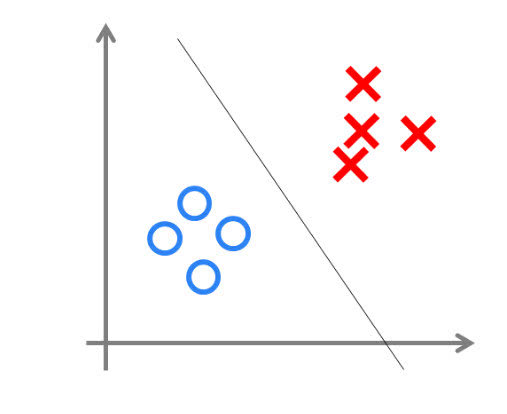2.4 单层神经网络表示
单层多感知器神经网络图示如下：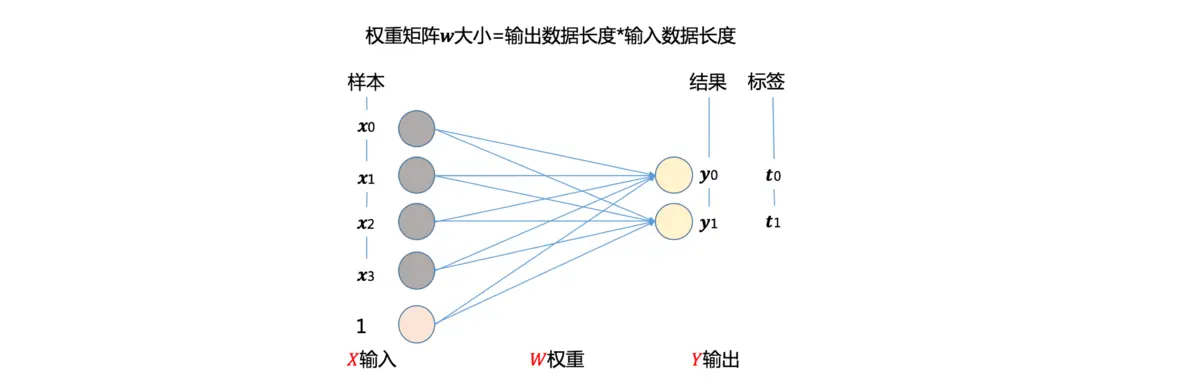其中数据表示如下：
输入数据向量:

X

→

=

[

x

0

x

1

…

x

n

]

(矩阵表示为：X)输出数据向量：

Y

→

=

[

y

0

y

1

…

y

m

]

(矩阵表示为：Y)权重矩阵：

W

=

[

ω

00

ω

01

…

ω

0

n

ω

10

ω

11

…

ω

1

n

…

…

…

…

ω

m

0

ω

m

1

…

ω

m

n

]

（n是输入特征数据长度，m数输出特征数据长度） （权重的每一行对应着一个感知器的权重，m行就意味着m个感知器）
2.5 单层神经网络训练算法
单层神经网络训练依据是基于如下目标：   就是找到一组感知器的权重，使得这组感知器的输出

Y

Y

与期望输出

Y

ˉ

\bar{Y}

之间的误差最小。

第一步：初始化一个随机权重矩阵（用来训练）； 第二步：输入特征数据

X

X

计算每个感知器(

m

m

个感知器)的输出

y

i

(

i

=

1

,

2

,

…

,

m

)

y_i(i=1,2,\dots,m)

，每个感知器的权重对应权重矩阵

W

W

中的一行，多个感知的输出就是输出向量

Y

Y

第三步：计算感知器输出向量

Y

Y

与样本期望输出

Y

ˉ

\bar{Y}

之间的误差。 第四步：根据计算的误差，计算权重矩阵的更新梯度。 第五步：用更新梯度，更新权重矩阵。 第六步：然后从第二步反复执行，直到训练结束（训练次数根据经验自由确定）

2.6 单层神经网络中的计算公式表示
在上面描述的训练过程中，有两个主要的计算公式：

感知器输出计算。权重的更新计算（核心是计算更新梯度）。

其中权重梯度的计算有两个依据：

误差的度量标准：损失函数的定义；误差最小：损失函数极小值计算。

根据这两个依据，我们可以列出单层神经网络的计算公式如下：
单层多感知器的计算输出公式：

Y

T

=

W

∙

X

T

+

W

b

Y^T=W\bullet X^T+W_b

X

：

输

入

特

征

数

据

，

使

用

行

向

量

表

示

.

X \ \ ：输入特征数据，使用行向量表示.

W

b

：

表

示

加

权

求

和

的

偏

置

项

W_b：表示加权求和的偏置项

。

如果考虑激活函数，则计算输出公式为：

Y

T

=

f

a

c

t

i

v

i

t

y

(

W

∙

X

T

+

W

b

)

Y^T=f_{activity}(W\bullet{X^T}+W_b)

单层多感知器的权重计算公式：

W

n

e

w

=

W

o

l

d

−

η

∗

∇

W

W_{new}=W_{old}-\eta\ast\nabla_{W}

w

i

n

e

w

=

w

i

o

l

d

−

η

∗

∇

w

i

w_i^{new}=w_i^{old}-\eta\ast\nabla_{w_i}

i

\ \ \ \ \ i

：表示第i个感知器

η

\ \ \ \ \ \eta

：表示学习率，用来控制训练速度。

∇

w

i

\nabla_{w_i}

：表示更新梯度（因为误差最小，是梯度下降，所以梯度更新是减去(-)梯度），梯度使用损失函数的导数，表示如下：

∇

w

i

=

∂

E

(

w

i

)

∂

w

i

\nabla_{w_i}=\frac{\partial{E{(w_i)}}}{\partial{w_i}}


展开全文• 单层神经网络线性回归A neural network is a powerful tool often utilized in Machine Learning because neural networks are fundamentally very mathematical. We will use our basics of Linear Algebra and ...

单层神经网络线性回归
A neural network is a powerful tool often utilized in Machine Learning because neural networks are fundamentally very mathematical. We will use our basics of Linear Algebra and NumPy to understand the foundation of Machine Learning using Neural Networks.
神经网络是机器学习中经常使用的强大工具，因为神经网络从根本上说是非常数学的。 我们将使用线性代数和NumPy的基础知识来理解使用神经网络进行机器学习的基础。
Our article is a showcase of the application of Linear Algebra and, Python provides a wide set of libraries that help to build our motivation of using Python for machine learning.
我们的文章展示了线性代数的应用，Python提供了广泛的库，有助于建立我们使用Python进行机器学习的动机。
The figure is showing a neural network with multi-input and one output node.
该图显示了一个具有多输入和一个输出节点的神经网络。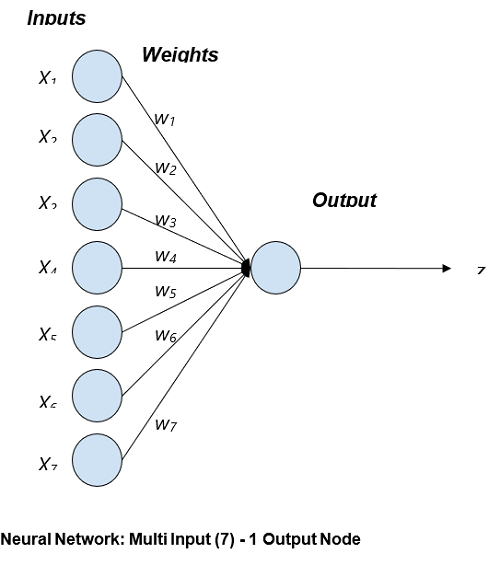Input to the neural network is X1, X2,  X3……... Xn and their corresponding weights are w1, w2, w3………..wn respectively. The output z is a tangent hyperbolic function for decision making which has input as the sum of products of Input and Weight.
输入到神经网络的是X 1 ， X 2 ， X 3 ……... X n ，它们的相应权重分别是w 1 ， w 2 ，w 3 ………..w n 。 输出z是决策的切线双曲函数，其输入为输入与权重的乘积之和。
Mathematically,  z = tanh(∑ Xiwi)
数学上z = tanh(∑ X i w i )
Where tanh( ) is an tangent hyperbolic function (Refer article Linear Algebra | Tangent Hyperbolic Function) because it is one of the most used decision-making functions.
其中tanh()是切线双曲函数(请参阅线性代数|切线双曲函数)，因为它是最常用的决策函数之一。
So for drawing this mathematical network in a python code by defining a function neural_network( X, W). Note: The tangent hyperbolic function takes input within the range of 0 to 1.
因此，通过定义函数Neuro_network(X，W)以python代码绘制此数学网络。 注意：切线双曲函数的输入范围为0到1。
Input parameter(s): Vector X and W
输入参数：向量X和W
Return: A value ranging between 0 and 1, as a prediction of the neural network based on the inputs.
返回值：一个介于0到1之间的值，作为基于输入的神经网络的预测。
Application:
应用：
Machine Learning 机器学习 Computer Vision 计算机视觉 Data Analysis 数据分析 Fintech 金融科技
单层神经网络的Python程序 (Python program for Uni - Layer Neural Network)
#Linear Algebra and Neural Network
#Linear Algebra Learning Sequence

import numpy as np

#Use of np.array() to define an Input Vector
inp = np.array([0.323, 0.432, 0.546, 0.44, 0.123, 0.78, 0.123])
print("The Vector A : ",inp)

#defining Weight Vector
weigh = np.array([0.3, 0.63, 0.99, 0.89, 0.50, 0.66, 0.123])
print("\nThe Vector B : ",weigh)

#defining a neural network for predicting an output value
def neural_network(inputs, weights):
wT = np.transpose(weights)
elpro = wT.dot(inputs)

#Tangent Hyperbolic Function for Decision Making
out = np.tanh(elpro)
return out

outputi = neural_network(inp,weigh)

#printing the expected output
print("\nExpected Output of the given Input data and their respective Weight : ", outputi)


Output:
输出：
The Vector A :  [0.323 0.432 0.546 0.44  0.123 0.78  0.123]

The Vector B :  [0.3   0.63  0.99  0.89  0.5   0.66  0.123]

Expected Output of the given Input data and their respective Weight :  0.9556019596251646


翻译自: https://www.includehelp.com/python/uni-layer-neural-network.aspx

单层神经网络线性回归

展开全文python 机器学习 深度学习
• 【火炉炼AI】深度学习002-构建并训练单层神经网络模型 (本文所使用的Python库和版本号: Python 3.6, Numpy 1.14, scikit-learn 0.19, matplotlib 2.2 ) 前面我们介绍了神经网络的基本结构单元-感知器，现在我们再升...
【火炉炼AI】深度学习002-构建并训练单层神经网络模型
(本文所使用的Python库和版本号: Python 3.6, Numpy 1.14, scikit-learn 0.19, matplotlib 2.2 )
前面我们介绍了神经网络的基本结构单元-感知器，现在我们再升一级，看看神经网络的基本结构和训练方法。

1. 单层神经网络
单层神经网络由一个层次中的多个神经元组成，总体来看，单层神经网络的结构是：一个输入层，一个隐含层，一个输出层。如下为结构示意图。图中描述的是前向网络，但其反向传播网络的结构也是一样的。蓝色方框表示输入层，绿色圆圈表示隐含层，输出层没有绘制出来。图片来源于2017/7/20 朱兴全教授学术讲座观点与总结第二讲：单个神经元/单层神经网络。
也可以从下图中看出单层神经网络的基本结构：那么从代码上怎么创建单层神经网络，并对其进行训练呢？
1.1 加载数据集
首先我们加载数据集，该数据集很简单，包含16行，四列，前两列是double型数据，构成了特征列，后两列是整数（0或1），构成了标签列。加载方式很简单，前面讲过多次，此处只把该数据集中特征列的分布情况绘制出来。如下1.2 建立模型并训练
数据集准备完成后， 就需要构建单层神经网络模型，并对其进行训练。
# 构建单层NN模型，该模型的隐含层含有两个感知器
import neurolab as nl
x_min, x_max = dataset_X[:,0].min(), dataset_X[:,0].max()
y_min, y_max = dataset_X[:,1].min(), dataset_X[:,1].max()
single_layer_net = nl.net.newp([[x_min, x_max], [y_min, y_max]], 2) # 隐含层含有两个神经元
# 所以本单层NN模型含有两个输入神经元，两个隐含层神经元，两个输出神经元
cost = single_layer_net.train(dataset_X, dataset_y, epochs=50, show=2, lr=0.01)
# 训练该单层NN模型，50个回合，每2个回合显示一下训练结果，学习速率为0.01

-------------------------------------输---------出--------------------------------
Epoch: 2; Error: 7.5; Epoch: 4; Error: 7.0; Epoch: 6; Error: 4.0; Epoch: 8; Error: 4.0; Epoch: 10; Error: 4.0; Epoch: 12; Error: 4.0; Epoch: 14; Error: 4.0; Epoch: 16; Error: 4.0; Epoch: 18; Error: 4.0; Epoch: 20; Error: 4.0; Epoch: 22; Error: 4.0; Epoch: 24; Error: 4.0; Epoch: 26; Error: 4.0; Epoch: 28; Error: 4.0; Epoch: 30; Error: 4.0; Epoch: 32; Error: 4.0; Epoch: 34; Error: 4.0; Epoch: 36; Error: 4.0; Epoch: 38; Error: 4.0; Epoch: 40; Error: 4.0; Epoch: 42; Error: 4.0; Epoch: 44; Error: 4.0; Epoch: 46; Error: 4.0; Epoch: 48; Error: 4.0; Epoch: 50; Error: 4.0; The maximum number of train epochs is reached
--------------------------------------------完-------------------------------------
可以看出在第6个回合时，cost就达到最低，并不再变化，表示已经收敛，再提高训练回合数也用处不大。将Error变化图绘制出来可以得到：1.3 使用训练好的模型来预测新样本
训练后的模型肯定是要用它来预测新样本，期望它能对从来没有见过的新样本也能得到理想的结果。
预测的代码为：
# 用训练好的模型来预测新样本
new_samples=np.array([[0.3, 4.5],
[4.5, 0.5],
[4.3, 8]])
print(single_layer_net.sim(new_samples))

-------------------------------------输---------出--------------------------------
[[0. 0.] [1. 0.] [1. 1.]]
--------------------------------------------完-------------------------------------
单单从结果上来看，我们成功的构建了单层NN模型并对其进行训练，通过训练后的模型来成功预测了新样本，一般的，一个深度学习模型流程就是这样的。
单层神经网络模型虽然结构简单，训练很快，比较适合一些简单问题，对于复杂一些的问题，这个模型就会力不从心，并且，有时我们的优化方法并不一定能够找到所希望的优化参数，也找不到所需要的拟合函数，由于模型比较简单，难以学习到复杂的内在机理，很容易产生欠拟合。
########################小**********结###############################
1，单层神经网络模型结构简单，训练耗时短，能够解决的问题也相对比较简单，对于比较复杂的问题会出现欠拟合，故而应用上受到一定限制，目前直接使用单层神经网络模型的情况非常少。
#################################################################

注：本部分代码已经全部上传到（我的github）上，欢迎下载。
参考资料:
1, Python机器学习经典实例，Prateek Joshi著，陶俊杰，陈小莉译
展开全文神经网络 深度学习 感知器 模型训练
• 本实验利用Python，搭建了一个用于识别猫的简易神经网络，最终实现在测试集上的准确率在70%以上。 实验环境： python中numpy、matplotlib、h5py和skimage库 训练样本： 209张64*64的带标签图片 测试样本： 50张64*64...
本实验利用Python，搭建了一个用于识别猫的单神经元神经网络，最终实现在测试集上的准确率在70%以上。
实验环境： python中numpy、matplotlib、h5py和skimage库
import numpy as np
import matplotlib.pyplot as plt  # 用于画图
import h5py  # 用于加载训练数据集
import skimage.transform as tf  # 用于缩放图片

训练样本： 209张64*64的带标签图片
测试样本： 50张64*64的带标签图片
关于本实验中所用数据集与完整代码详见： https://github.com/PPPerry/AI_projects/tree/main/1.cats_identification
构建的神经网络模型如下：基于该神经网络模型，代码实现如下：
首先，对数据集数据进行预处理。
def load_dataset():
"""
加载数据集数据
:return: 训练数据与测试数据的相关参数
"""
train_dataset = h5py.File("datasets/train_catvnoncat.h5", "r")
train_set_x_orig = np.array(train_dataset["train_set_x"][:])  # 提取训练数据的特征数据，格式为(样本数, 图片宽, 图片长, 3个RGB通道)
train_set_y_orig = np.array(train_dataset["train_set_y"][:])  # 提取训练数据的标签数据，格式为(样本数, )

test_dataset = h5py.File("datasets/test_catvnoncat.h5", "r")
test_set_x_orig = np.array(test_dataset["test_set_x"][:])  # 提取测试数据的特征数据
test_set_y_orig = np.array(test_dataset["test_set_y"][:])  # 提取测试数据的标签数据

classes = np.array(test_dataset["list_classes"][:])  # 提取标签，1代表是猫，0代表不是猫

train_set_y_orig = train_set_y_orig.reshape((1, train_set_y_orig.shape))  # 统一类别数组格式为(1, 样本数)
test_set_y_orig = test_set_y_orig.reshape((1, test_set_y_orig.shape))

return train_set_x_orig,  train_set_y_orig, test_set_x_orig, test_set_y_orig, classes

train_set_x_orig,  train_set_y, test_set_x_orig, test_set_y, classes = load_dataset()  # 加载数据集数据

m_train = train_set_x_orig.shape  # 训练样本数
m_test = test_set_x_orig.shape  # 测试样本数
num_px = test_set_x_orig.shape  # 正方形图片的长/宽

train_set_x_flatten = train_set_x_orig.reshape(train_set_x_orig.shape, -1).T  # 将样本数据进行扁平化和转置，格式为(图片数据, 样本数)
test_set_x_flatten = test_set_x_orig.reshape(test_set_x_orig.shape, -1).T

train_set_x = train_set_x_flatten/255.  # 标准化处理，使所有值都在[0, 1]范围内
test_set_x = test_set_x_flatten/255.

其次，构造神经网络中用到的相应函数。 其中，前向传播的公式为

A

=

σ

(

w

T

X

+

b

)

=

(

a

(

1

)

,

a

(

2

)

,

⋯

,

a

(

m

−

1

)

,

a

(

m

)

)

J

=

−

1

m

∑

i

=

1

m

l

o

g

(

a

(

i

)

)

+

(

1

−

y

(

i

)

)

l

o

g

(

1

−

a

(

i

)

)

\begin{array}{c} A=\sigma(w^TX+b)=(a^{(1)},a^{(2)},\cdots,a^{(m-1)},a^{(m)})\\ \\ J = -\frac{1}{m}\sum^m_{i=1}log(a^{(i)})+(1-y^{(i)})log(1-a^{(i)}) \end{array}

反向传播的公式为

∂

J

∂

w

=

1

m

X

(

A

−

Y

)

T

∂

J

∂

b

=

1

m

∑

i

=

1

m

(

a

(

i

)

−

y

(

i

)

)

\begin{array}{c} \frac{\partial J}{\partial w} = \frac{1}{m}X(A-Y)^T\\ \\ \frac{\partial J}{\partial b} = \frac{1}{m}\sum^m_{i=1}(a^{(i)}-y^{(i)}) \end{array}

def sigmoid(z):
"""
sigmod函数实现
:param z: 数值或一个numpy数组
:return: [0, 1]范围数值
"""
s = 1 / (1 + np.exp(-z))
return s

def initialize_with_zeros(dim):
"""
初始化权重数组w和偏置b为0
:param dim: 权重值的数量
:return:
w: 权重数组
b: 偏置bias
"""
w = np.zeros((dim, 1))
b = 0
return w, b

def propagate(w, b, X, Y):
"""
实现正向传播和反向传播，分别计算出成本与梯度
:param w: 权重数组
:param b: 偏置
:param X: 图片的特征数据
:param Y: 图片的标签数据
:return:
cost: 成本
dw: w的梯度
db：b的梯度
"""
m = X.shape

# 前向传播
A = sigmoid(np.dot(w.T, X) + b)
cost = -np.sum(Y * np.log(A) + (1 - Y) * np.log(1 - A)) / m

# 反向传播
dZ = A - Y
dw = np.dot(X, dZ.T) / m
db = np.sum(dZ) / m

# 梯度保存在字典中
"dw": dw,
"db": db
}

def optimize(w, b, X, Y, num_iterations, learning_rate, print_cost=False):
"""
梯度下降算法更新参数
:param w: 权重数组
:param b: 偏置bias
:param X: 图片的特征数据
:param Y: 图片的标签数据
:param num_iterations: 优化迭代次数
:param learning_rate: 学习率
:param print_cost: 为真时，每迭代100次，打印一次成本
:return:
params: 优化后的w和b
costs: 每迭代100次，记录一次成本
"""
costs = []

for i in range(num_iterations):
grads, cost = propagate(w, b, X, Y)

# 梯度下降
w = w - learning_rate * dw
b = b - learning_rate * db

# 记录成本变化
if i % 100 == 0:
costs.append(cost)
if print_cost:
print("优化%d次后成本是：%f" % (i, cost))

params = {
"w": w,
"b": b
}

return params, costs

def predict(w, b, X):
"""
预测函数，判断是否为猫
:param w: 权重数组
:param b: 偏置bias
:param X: 图片的特征数据
:return:
Y_predicition: 预测是否为猫，返回值为0或1
p: 预测为猫的概率
"""
m = X.shape
Y_prediction = np.zeros((1, m))

p = sigmoid(np.dot(w.T, X) + b)

for i in range(p.shape):
if p[0, i] >= 0.5:
Y_prediction[0, i] = 1

return Y_prediction, p

最终，组合以上函数，构建出最终的神经网络模型函数。
def model(X_train, Y_train, X_test, Y_test, num_iterations=2001, learning_rate=0.5, print_cost=False):
"""
最终的神经网络模型函数
:param X_train: 训练样本的特征数据
:param Y_train: 训练样本的标签数据
:param X_test: 测试样本的特征数据
:param Y_test: 测试样本的标签数据
:param num_iterations: 优化迭代次数
:param learning_rate: 学习率
:param print_cost: 为真时，每迭代100次，打印一次成本
:return:
d: 返回相关信息的字典
"""
w, b = initialize_with_zeros(X_train.shape)  # 初始化参数

parameters, costs = optimize(w, b, X_train, Y_train, num_iterations, learning_rate, print_cost)  # 训练参数
w = parameters["w"]
b = parameters["b"]

Y_prediction_train, p_train = predict(w, b, X_train)
Y_prediction_test, p_test = predict(w, b, X_test)

print("对训练数据的预测准确率为：{}%".format(100 - np.mean(np.abs(Y_prediction_train - Y_train)) * 100))
print("对测试数据的预测准确率为：{}%".format(100 - np.mean(np.abs(Y_prediction_test - Y_test)) * 100))

d = {
"costs": costs,
"Y_prediction_test": Y_prediction_test,
"Y_prediction_train": Y_prediction_train,
"w": w,
"b": b,
"learning_rate": learning_rate,
"num_iterations": num_iterations,
"p_train": p_train,
"p_test": p_test
}

return d

现在调用上面的模型函数对我们最开始加载的数据进行训练。
d = model(train_set_x, train_set_y, test_set_x, test_set_y, num_iterations=2001, learning_rate=0.005, print_cost=True)

输出：优化2000次后的成本是0.1356。对训练数据的准确率为99%左右，对测试数据的准确率为70%左右。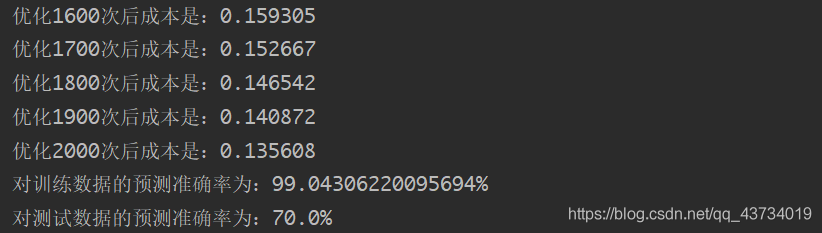试验模型预测功能：
查看训练集和测试集中图片及其对应的预测结果
def show_predict(index, prediction=np.hstack((d["Y_prediction_train"], d["Y_prediction_test"])),
data=np.hstack((train_set_x, test_set_x)), origin=np.hstack((train_set_y, test_set_y)),
px=num_px, p=np.hstack((d["p_train"], d["p_test"]))):
if index >= prediction.shape:
print("index超出数据范围")
return
plt.imshow(data[:, index].reshape((px, px, 3)))
plt.show()
print("这张图的标签是" + str(origin[0, index]) + "，预测分类是" + str(int(prediction[0, index])) + "，预测概率是" + str(p[0, index]))

return

以第19张图片为例：输出：这张图的标签是1，预测分类是1，预测概率是0.93。
查看自己输入的图片及其对应的预测结果
# 预测自己的图片
# 在同目录下创建一个文件夹images,把你的任意图片改名成my_image1.jpg后放入文件夹
my_image = "my_image1.jpg"

my_image = tf.resize(image, (num_px, num_px), mode='reflect').reshape((1, num_px*num_px*3)).T
my_prediction, my_p = predict(d["w"], d["b"], my_image)

plt.imshow(image)
plt.show()
print("预测分类是" + str(int(my_prediction[0, 0])) + "，预测概率是" + str(my_p[0, 0]))

以下图为例：输出：预测分类是1，预测概率是0.89。
进一步实验得到的结论：
成本随迭代次数增加时的变化情况
# 绘制成本随迭代次数增加时的变化情况
costs = np.squeeze(d['costs'])  # 将表示向量的数组转换为秩为1的数组，便于matplotlib库函数画图
plt.plot(costs)
plt.ylabel('cost')
plt.xlabel('iterations (per hundreds)')
plt.title("Learning rate =" + str(d["learning_rate"]))
plt.show()结论：训练次数越多，成本越小，预测结果越精确。
不同学习率下成本随迭代次数增加时的变化情况
# 绘制在不同学习率下成本随迭代次数增加时的变化情况
learning_rates = [0.01, 0.001, 0.0001]
models = {}
for i in learning_rates:
models[str(i)] = model(train_set_x, train_set_y, test_set_x, test_set_y, num_iterations=2001, learning_rate=i,
print_cost=False)

for i in learning_rates:
plt.plot(np.squeeze(models[str(i)]["costs"]), label=str(models[str(i)]["learning_rate"]))

plt.ylabel('cost')
plt.xlabel('iterations (per hundreds)')
plt.show()结论：选择一个正确的学习率很重要，不一定越大越好。设置不合理，神经网络有可能永远都不能下降到损失函数的最小值处；相反，设置合理，可以使神经网络的收敛达到很好的效果。
结束语：
实验过程中，一定要搞清楚变量的维度，否则做数据处理、写数组操作时会容易出现问题。数据集、图片等需要和代码在同一目录下。本实验几乎基于纯Python的numpy库函数进行神经网络模型的搭建，没有使用任何机器学习框架，重在理解数学原理及意义。在后续的实验中，我会对TensorFlow等经典框架进行学习与运用。相关代码可能会不断更新改进，以github中的代码为准。这是我在系统学习人工智能中实现的第一个人工智能程序，后续还会不断更新系列实验。写博客以督促自己不能总摸鱼233，希望自己能在神经网络这条路上走的尽可能远一些。
展开全文python 机器学习 深度学习
• 使用python手写实现单层神经网络[本质上学习logistic 回归的系数]。单层：有参数的一层；输入不算网络层。 网络用途 或者说应用场景：使用单层神经网络来识别一张图片是否是猫咪的图片。 数学表示 给定一张...深度学习
• 人工AI — 神经网络构建初步 ...我们将构建一个没有隐藏层或感知器的单层神经网络。它由一个包含训练示例、突触或权重以及神经元的输入层和一个含有正确答案的输出层组成。神经网络图形如下所示： 此外，需要了解一python 机器学习 深度学习
• 学习使用了官方文档的样例，单层神经网络训练MNIST数据集，在此做一个简单的总结与记录。官方中文档入口。  内容主要都写在注释里了。有一个问题在这里注明一下：  训练迭代次数过多后，准确率会断崖式跌落至9.8...Python TensorFlow 深度学习
• 一般神经网络的训练包括几个重要的步骤，数据准备、初始化权重、激活函数、前向计算、损失函数、计算损失、反向计算、更新参数，直到收敛或达到终止条件。 本篇介绍神经网络在iris数据集上完成多分类的任务。 程序头...深度学习
• 一. 线性多分类问题 1 提出问题 我们解决了公元前的楚汉相争的问题，现在看一下公元220年前后的三国问题。...从视觉上判断是线性可分的，所以我们使用单层神经网络即可 2.输入特征是两个，X1...深度学习 机器学习 线性分类 线性多分类
• 从联立后的式子可以看出，虽然神经网络引入了隐藏层，却依然等价于一个单层神经网络：其中输出层权重参数为 ，偏差参数为 。不难发现，即便再添加更多的隐藏层，以上设计依然只能与仅含输出层的单层神经网络等价。 ...
• 大多数人在学一门新的编程语言时，通常要写的第一个程序是输出“Hello World”，在人工智能中，手写数字识别问题就是“Hello World”，本文将简要介绍如何使用TensorFlow构造一个简单的单层神经网络，来解决手写数字...tensorflow 手写数字识别
• 1.引子　 ...　感知器是当时首个可以学习的人工神经网络。Rosenblatt现场演示了其学习识别简单图像的过程，在当时的社会引起了轰动。 　人们认为已经发现了智能的奥秘，许多学者和科研机构纷纷投...
• 本篇文章，我们开始介绍最简单的神经网络结构，感知器，在了解原理的基础上，下篇博客我们代码实现一个单层感知器： 感知器： 人工神经网络的第一个里程碑是感知机perceptron， 这个名字其实有点误导， 因为它...
• 本文综合整理常用的神经网络，包括生物神经网络人工神经网络、卷积神经网络、循环神经网络、生成对抗网络；参考了许多高校的课程、论文、博客和视频等。文章的结构是先进行概念了解，然后结合图片、结构图、一步...深度学习
• 神经元采用最简单的简化MP（麦卡洛克-皮茨）模型，模型表示如下： 在鸢尾花分类的例子里，输入为x0,x1,x2,x3以及偏置项b。我们可以定义鸢尾花特征有四个维度，每个维度分别对应一个输入x。 简化模型可以表示为y=x0*...
• #!/usr/bin/env python2 # -*- coding: utf-8 -*- import tensorflow as tf from tensorflow.examples.tutorials.mnist import input_data mnist = input_data.read_data_sets("MNIST_data/", one_hot=True) ...tensorflow
• 单层感知器（Single Layer Perceptron）是最简单的神经网络。它包含输入层和输出层，而输入层和输出层是直接相连的。
• 构造一个4个输出的单层网络， import mxnet . ndarray as nd from mxnet . gluon import nn layer1 = nn . Dense ( 4 ) #权重默认为平均分布的[-0.7,0.7]，4是指输出的维度的第2维的值 print ( layer1 )......